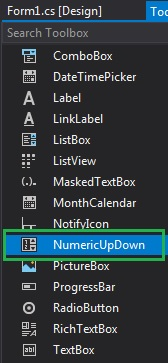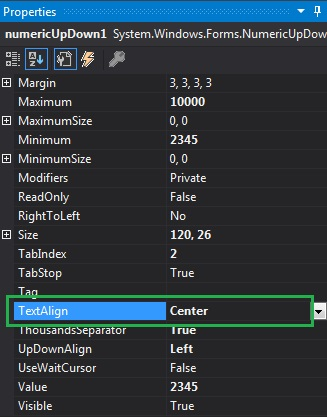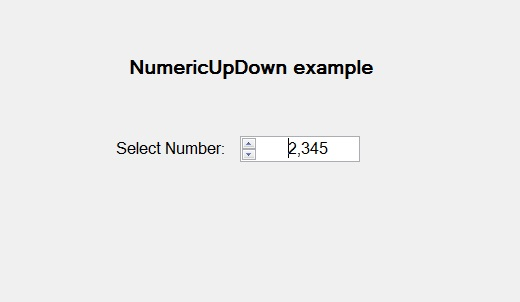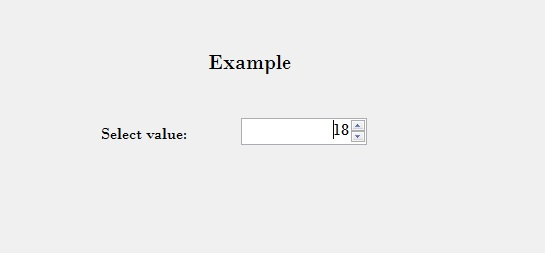# How to set the Alignment of the Text in NumericUpDown in C#?

• Last Updated : 29 Jul, 2019

In Windows Forms, NumericUpDown control is used to provide a Windows spin box or an up-down control which displays the numeric values. Or in other words, NumericUpDown control provides an interface which moves using up and down arrow and holds some pre-defined numeric value. In NumericUpDown control, you can set the alignment of the text present in the up-down control using TextAlign Property. This property contains three different values which are defined under HorizontalAlignment enum and the values are:

• Center: It aligns the text in the center of the NumericUpDown control.
• Left: It aligns the text on the left side of the NumericUpDown control.
• Right: It aligns the text on the right side of the NumericUpDown control.

The default value of this property is left. You can set this property in two different ways:

1. Design-Time: It is the easiest way to set the alignment of the text in the NumericUpDown as shown in the following steps:

• Step 1: Create a windows form as shown in the below image:
Visual Studio -> File -> New -> Project -> WindowsFormApp• Step 2: Next, drag and drop the NumericUpDown control from the toolbox on the form as shown in the below image:• Step 3: After drag and drop you will go to the properties of the NumericUpDown and set the alignment of the text in the NumericUpDown as shown in the below image:Output:2. Run-Time: It is a little bit trickier than the above method. In this method, you can set the alignment of the text in the NumericUpDown control programmatically with the help of given syntax:

`public System.Windows.Forms.HorizontalAlignment TextAlign { get; set; }`

Here, HorizontalAlignment represents the value of this property. If the value of this property does not belong to the HorizontalAlignment enum, then it will throw InvalidEnumArgumentException. The following steps show how to set the alignment of the text in the NumericUpDown dynamically:

• Step 1: Create a NumericUpDown using the NumericUpDown() constructor is provided by the NumericUpDown class.
```// Creating a NumericUpDown
NumericUpDown n = new NumericUpDown();
```
• Step 2: After creating NumericUpDown, set the TextAlign property of the NumericUpDown provided by the NumericUpDown class.
```// Setting the TextAlign
n.TextAlign = HorizontalAlignment.Right;
```
• Step 3: And last add this NumericUpDown control to the form using the following statement:
```// Adding NumericUpDown control on the form
 `using` `System;``using` `System.Collections.Generic;``using` `System.ComponentModel;``using` `System.Data;``using` `System.Drawing;``using` `System.Linq;``using` `System.Text;``using` `System.Threading.Tasks;``using` `System.Windows.Forms;`` ` `namespace` `WindowsFormsApp44 {`` ` `public` `partial` `class` `Form1 : Form {`` ` `    ``public` `Form1()``    ``{``        ``InitializeComponent();``    ``}`` ` `    ``private` `void` `Form1_Load(``object` `sender, EventArgs e)``    ``{`` ` `        ``// Creating and setting the``        ``// properties of the labels``        ``Label l1 = ``new` `Label();``        ``l1.Location = ``new` `Point(348, 61);``        ``l1.Size = ``new` `Size(215, 25);``        ``l1.Text = ``"Example"``;``        ``l1.Font = ``new` `Font(``"Bodoni MT"``, 16);``        ``this``.Controls.Add(l1);`` ` `        ``Label l2 = ``new` `Label();``        ``l2.Location = ``new` `Point(242, 136);``        ``l2.Size = ``new` `Size(103, 20);``        ``l2.Text = ``"Select value:"``;``        ``l2.Font = ``new` `Font(``"Bodoni MT"``, 12);``        ``this``.Controls.Add(l2);`` ` `        ``// Creating and setting the``        ``// properties of NumericUpDown``        ``NumericUpDown n = ``new` `NumericUpDown();``        ``n.Location = ``new` `Point(386, 130);``        ``n.Size = ``new` `Size(126, 26);``        ``n.Font = ``new` `Font(``"Bodoni MT"``, 12);``        ``n.Value = 18;``        ``n.Minimum = 18;``        ``n.Maximum = 30;``        ``n.Increment = 1;``        ``n.TextAlign = HorizontalAlignment.Right;`` ` `        ``// Adding this control``        ``// to the form``        ``this``.Controls.Add(n);``    ``}``}``}`